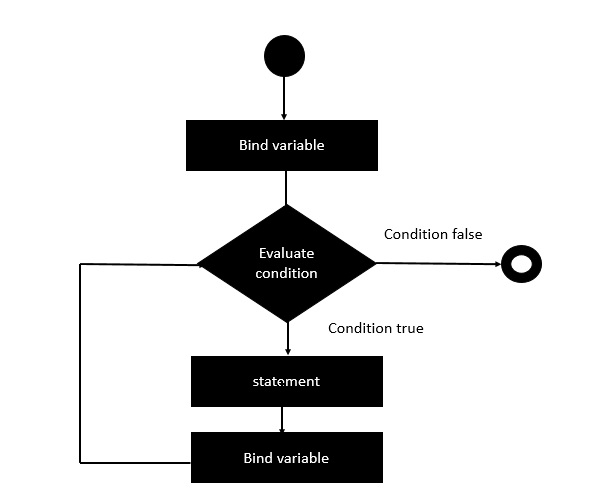# Clojure - Loop Statement

The loop special form is not like a ‘for’ loop. The usage of loop is the same as the let binding. However, loop sets a recursion point. The recursion point is designed to use with recur, which means loop is always used with recur. To make a loop happen, the number of arguments (arity) specified for recurs must coincide with the number of bindings for the loop. That way, recur goes back to the loop.

## Syntax

Following is the general syntax of the loop statement.

```loop [binding]
(condition
(statement)
(recur (binding)))
```

Following is the diagrammatic representation of this loop.## Example

Following is an example of a ‘for-in’ statement.

```(ns clojure.examples.hello
(:gen-class))

;; This program displays Hello World
(defn Example []
(loop [x 10]
(when (> x 1)
(println x)
(recur (- x 2)))))
(Example)
```

In the above example, we are first binding the value of ‘x’ to 10 using the loop statement. We then use the when condition clause to see if the value of ‘x’ is less than 1. We then print the value of ‘x’ to the console and use the recur statement to repeat the loop. The loop is repeated after the value of ‘x’ is decremented by 2.

## Output

The above code produces the following output.

```10
8
6
4
2
```
clojure_loops.htm